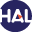# Volume 6 N°2 (2009)

Latest addition : 6 December 2009.

• ### A model for unsteady mixed flows in non uniform closed water pipes and a well-balanced finite volume

6 December 2009,

Full Title: A model for unsteady mixed flows in non uniform closed water pipes and a well-balanced finite volume

Abstract:

We present the derivation of a new unidirectional model for unsteady mixed flows in non uniform closed water pipes. We introduce a local reference frame to take into account the local perturbation caused by the changes of section and slope. Then an asymptotic analysis is performed to obtain a model for free surface flows and another one for pressurized flows.

By coupling these models through the transition points using a common set of variables and a suitable pressure law, we obtain a simple formulation called PFS-model close to the shallow water equations with source terms. It takes into account the changes of section and the slope variation in a continuous way through transition points. Transition points between the two types of flows are treated as a free boundary associated to a discontinuity of the gradient of pressure.

The numerical simulation is performed by making use of a VFRoe scheme that we adapted to take into account geometrical source terms in the convection matrix. By a suitable definition of the convection matrix, we obtain an exactly well-balanced scheme.

Finally some numerical tests are presented.Voir sur HAL : https://hal.archives-ouvertes.fr/hal-00342745

• ### A nonlinear finite volume scheme satisfying maximum and minimum principles for diffusion operators

6 December 2009,

Full Title: A nonlinear finite volume scheme satisfying maximum and minimum principles for diffusion operators

Abstract:

We introduce a new finite volume method for highly anisotropic diffusion operators on triangular cells.

The main idea is to calculate the gradient using a nonlinear scheme. For parabolic problems, the resulting global matrix is a strictly diagonally dominant M-Matrix without geometrical constraints on the mesh and restrictive conditions on the anisotropy ratio. We verify the accuracy of the method by comparing our computed solutions with analytical solutions.

The efficiency of the algorithm is demonstrated by comparing it with numerical schemes which do not satisfy discrete minimum and maximum principles.Voir sur HAL : https://hal.archives-ouvertes.fr/hal-01116968